# Design and Implementation of Walking Beam Manipulator in Automatic Production Line Based on PLC

Design and Implementation of Walking Beam Manipulator in Automatic Production Line Based on PLC
School of Mechanical & Automotive Engineering, Anhui Technical College of Water Resources and Hydroelectric Power, Hefei 231603, China

Corresponding Author Email:
zhangningasd@126.com
Page:
173-178
|
DOI:
https://doi.org/10.18280/jesa.520209
20 January 2019
|
Accepted:
6 April 2019
|
Published:
15 July 2019
| Citation

OPEN ACCESS

Abstract:

This paper analyzes the kinematic features of the walking beam manipulator in the semi-automatic production line of cracked connecting rods, examines the positioning accuracy between the manipulator and each workstation, and explores the dynamic response of the position servo controller. Next, the author designed a position control system for the production line based on the electric drive mode, and detailed the hardware scheme of the system. The position control system integrates a specially-designed algorithm, which combines feedforward control and trigonometric function. Finally, the proposed system was proved to achieve accurate control of sequential motion and position between the walking beam manipulator and each workstation, especially in high-speed operation.

Keywords:

walking beam manipulator, automatic production line, position servo system, proportional–integral–derivative (PID) control

1. Introduction

It is a complicated process to design and implement the control system for complex objects. The design of the control system, if based on traditional control modes, will face many functional and structural problems, including but not limited to high cost, long cycle and instability. Fortunately, the fieldbus control system provides an electric drive mode that can accurately regulate complex targets like the position of a fast-moving object. In this mode, the driving mechanism, consisting of a servo system and an alternating current (AC) servomotor, is regulated by programmable logic controller (PLC) and a reasonable position accuracy control algorithm. This control mode is much more accurate and stable than the traditional hydraulic drive mode and pneumatic drive mode. As a result, the fieldbus control system is a suitable way to control semi-automatic and automatic production lines of cracked connecting rods, laying an important basis for large process control network of industrial automation and control systems [1-3].

In semi-automatic and automatic production lines of cracked connecting rods, the walking beam manipulator, which mediates the connecting rods between workstations, is a typical fast-moving object. The performance of the manipulator directly bears on the stability of the entire production line. To enhance the production quality, it is necessary to design a control system that properly regulates the motion of the walking beam manipulator. Therefore, this paper analyzes the kinematic features of the walking beam manipulator in the semi-automatic production line of cracked connecting rods, examines the positioning accuracy between the manipulator and each workstation, and explores the dynamic response of the position servo controller. Next, the author designed a position control system for the production line based on the electric drive mode, and detailed the hardware scheme of the system. The position control system integrates a specially-designed algorithm, which combines feedforward control and trigonometric function. Finally, the proposed system was proved to achieve accurate control of sequential motion and position between the walking beam manipulator and each workstation, especially in high-speed operation.

2. Design of Position Control System

The servo system [4, 5] is an automatic control mechanism capable of adjusting the position, azimuth and state of an object with dynamic changes in input values. The main task of the system is to achieve flexible and convenient control of the torque, speed and position of the driving device. To fulfil the task, the system needs to amplify, transform and regulate the power accurately as per the control command. In ideal situation, the servo system should operate stability despite variations in load, voltage or ambient temperature.

The position servo system, a.k.a. position control system , is a closed-loop automatic control system with feedback. By the definition of the servo system, the position servo system faces the task of precise position control, i.e. tracking the object motion in a rapid and accurate manner. As shown in Figure 1, a typical position servo system consists of a position control module, a servo drive module, a mechanical drive module and a position detection module.

## 1.png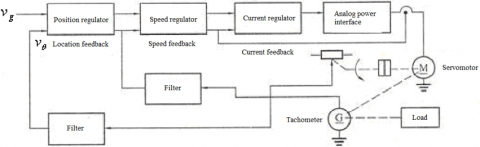Figure 1. Architecture of position servo system

In Figure 1, the output voltage vθ of potentiometer is linearly correlated with its position. The position regulator minimizes the deviation between position-given signal vg and position-feedback signal vθ, and sends the corrected signal to the speed regulator and current regulator. Then, the speed regulator adjusts the overshoot of the damping position, and the current regulator reduces the moment fluctuation, limits the maximum current, and speeds up the dynamic response. The harmonic signals in the output of the position or speed regulators are filtered out in the filter circuit. Next, the servomotor, driven by analog power interface, implements position servo control.

The control precision of position servo system is measured by positioning accuracy, i.e. the static error between the final position of the object and the position required in the system command (hereinafter referred to as the command speed). In the system, the speed regulator should cover a wide range of speeds and perform excellently in low-speed operation. The principle of speed control is illustrated in Figure 2 below.

## 2.png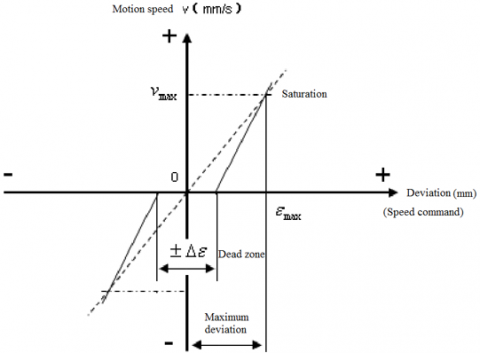Figure 2.  Principle of speed control

As shown in Figure 2, when the input command falls in the range of ±∆ε, the servomotor does not rotate or rotates unstably; when the input command reaches εmax, the servomotor rotates at the maximum speed vmax and enters the saturation state. Therefore, εmax/∆ε was taken as the range of speed control.

If it equals one pulse in static condition, the input command must surpass the minimum positioning accuracy ∆ε to kick off the position servo system. In other words, the speed control range should satisfy the following constraint:

$D \geq \frac { \varepsilon _ { \max } } { \Delta \varepsilon } = \frac { v _ { \max } } { K _ { h } \cdot \Delta \varepsilon }$  (1)

where Kh is open-loop gain of position loop. Eq. (1) shows that the positioning accuracy of the position servo system must satisfy:

$\Delta \varepsilon \geq \frac { v _ { \max } } { K _ { h } \cdot D }$   (2)

2.1 Hardware design

For the semi-automatic production line of cracked connecting rods, the position control system should be designed considering the sequential motion control and position accuracy control between the walking beam manipulator and each workstation. In light of the motion features of the manipulator, this subsection designs the hardware structure of the motion control system. The hardware design contains of PLC, motion control card, servo drive unit and AC servomotor. Figure 3 presents the transmission structure between the manipulator and each workstation under the electric drive mode.

## 3.png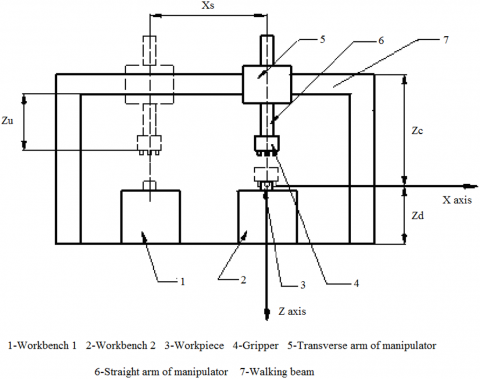Figure 3. The transmission structure between the manipulator and each workstation

In the target production line, the walking beam manipulator can move in both the x-direction and the z-direction. Below is a brief description of the manipulator operation in one cycle:

Step 1: The manipulator moves in the x-direction on the walking beam, the straight arm drops from the initial position to the down position Zd, the gripper closes, and the manipulator grabs the workpiece from workbench 1.

Step 2: The straight arm rises from the down position Zd to the up position Zu, the transverse arm moves along the walking beam opposite to the x-direction from Xs to the top of workbench 2, and the straight arm falls from the up position Zu back to the down position Zd.

Step 3: The gripper opens and places the connecting rod on the workbench, marking the end of the transmission process. Then, the straight arm rises from Zd to Zu, while the transverse arm moves along the x-direction from  Xs on the walking beam to the initial position, waiting for the operation in the next cycle.

Drawing on the integrated design of the most advanced position servo systems, this paper puts forward an intelligent position servo controller that integrates the control strategies of the position, speed and current loops into a double closed-loop servo system. The inner loop, as the main control loop, integrates current and speed controls, while the outer loop provides position/angle control. During the control process, the system converts the position control input into the command speed, and the servomotor is driven to move the object to the required position. The hardware scheme of the proposed intelligent position servo controller is shown in Figure 4 below.

## 4.png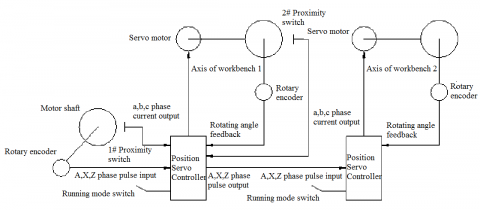Figure 4. The hardware scheme of the proposed intelligent position servo controller

In theory, the walking beam manipulator can act in coordination with each workstation, as long as the command is correct. However, there is always angle deviation or overshoot in the servo drive unit of each workstation, arising from the manufacturing deviation of the axle and the difference in the driving circuit parameters. The deviation could be random or systematic. Random deviations need no special treatment, because they offset each other in the long run. Since the workbench moves in round-trip at each workstation, systematic deviations, which are directional, often result in reverse clearance error.

The reverse clearance exists between the screw and nut pairs in the feed drive chain of the machine tool, which comes from the insufficient preload or abrasion of lead screw. The existence of reverse clearance dampens the positioning accuracy of the machine tool, which is a semi-closed-loop servo system, which in turn undermines the processing accuracy of the product. With the elapse of time, the reverse clearance will gradually increase with the wear-induced pair clearance [7, 8].

The reverse clearance can be measured in the following steps: First, moving a distance forward or backward in advance within the distance of the coordinate axis being measured, taking the desired stop position as the reference; then, moving a distance in the positive and negative directions of the axis as per the command; finally, measuring  the difference between the actual stop positions and the reference position.

2.2 Proportional–integral–derivative (PID) control algorithm

In the hardware design, the PLC, servo control system and servomotor provide a strong guarantee of the positioning stability and accuracy. However, the walking beam manipulator in the target system should not only achieve accurate positioning, but also make rapid, dynamic response. In the proposed servo positioning system, the position command was forwarded to the servo system by the fixed algorithm in the upper controller. This mode may cause out-of-step and position overshoot as the servo control system speeds up and slows down to execute the command. This calls for a robust algorithm to control the speed and position between the walking beam manipulator and each workstation. Here, the popular PID control algorithm [9, 10] is introduced to realize precise position control. The PID control diagram is detailed in Figure 5 below.

## 5.png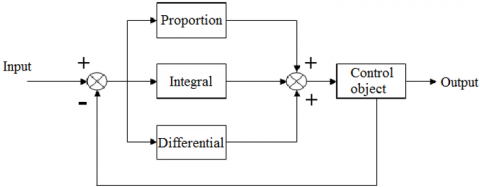Figure 5. The PID control diagram

Under the PID control, the control quantity is calculated by proportional, integral and differential operations.

The proportional control is the simplest form of control. The output signal of the controller is proportional to the input signal. The control law can be expressed as u(t)=Kpe(t), where u(t) is the output signal, e(t) is the input error signal, and Kp is the proportionality coefficient, while the transfer function can be described as G(s)=Kp.

In integral control, the output signal of the controller is proportional to the integral of the input error signal. The control law can be expressed as $u ( t ) = \frac { K _ { p } } { T _ { i } } \int e ( t ) d t$, while the transfer function can be described as  $G ( s ) = K _ { p } / T _ { i } S$, where Ti is the integral time constant.

The integral term is indispensable to the controller to eliminate steady-state error. The error of the integral term depends on the integral of time. However small the error, the integral term always increases with the elapse of time. Thus, the output signal of the controller will grow accordingly, and the steady-state error will be reduced all the way to zero. Hence, the proportional and integral controls are often combined into the proportion + integral controller, whose control law and transfer function are respectively $u ( t ) = K _ { p } \left[ e ( t ) + \frac { 1 } { T _ { i } } \int e ( t ) d t \right]$ and $G ( s ) = K _ { p } \left( 1 + 1 / T _ { i } s \right)$.

In differential control, the output signal of the controller is proportional to the differential of the input error signal. The control law and transfer function can be described as $u ( t ) = K _ { p } T _ { d } d e ( t ) / d t$ and $G ( s ) = K _ { p } T _ { d } s$, respectively.

Therefore, the control law and transfer function of the PID controller can be respectively described as $u ( t ) = K _ { p } \left[ e ( t ) + \frac { 1 } { T _ { i } } \int e ( t ) d t + T _ { d } \frac { d e ( t ) } { d t } \right.$ and $G ( s ) = K _ { p } \left( 1 + \frac { 1 } { T _ { i } s } + T _ { d } S \right)$. With properly selected parameters, the three control modes of the PID controller can work together to make the system operation fast, agile, stable and accurate.

The PID control varies with the control objects. Many different PID control schemes have been developed at home and abroad, ranging from model-based design , delayed feedback test  to dominant pole design . Nonetheless, these schemes are based on linear systems, and not applicable to nonlinear systems. As a result, many scholars have improved the PID control algorithm for nonlinear systems. Hua et al.  employed the neural network to simulate the nonlinear system for PID design. Yang et al.  constructed an adaptive PID controller model by least squares method. Li et al.  optimized PID control parameters for a polymer reactor, using the genetic algorithm. Yu et al.  proposed a two-level supervisory control strategy to revise the parameters of the PID controller.

Inspired by the relevant studies, this paper designs the position loop as a proportional control link to improve the control of the walking beam manipulator. By adjusting the proportional gain, it is possible to eliminate the overshoot in the position response of the system. In this way, a position controller was put forward based on speed and acceleration feedforward control, and the trigonometric function of acceleration and deceleration was used to control the algorithm. The feedforward controller assures the fast-dynamic response of the system, while the system accuracy is maintained by adjusting the proportional integral coefficient of the position loop. Figure 6 shows the structure of the feedforward control mechanism.

## 6.pngFigure 6. The structure of the feedforward control mechanism

The position feedforward control follows the control principle of forward difference. In each sampling period, the motion control card sends out the pulse command pc to the servo drive system:

$p _ { c } = K _ { p } \left( P _ { 0 } - P _ { b } \right) + K _ { f } \left( P _ { 1 } - P _ { 0 } \right)$.

where Kis the feedforward coefficient; P0 and P1 are the command positions in the current period and the next period, respectively. The pulse command is determined by the position error in the current period, and the interpolation point of the next sampling period.

In Figure 6, R(s) is the command position and C(s) is the position of the servomotor. When the speed regulator adopts proportional control, the closed-loop transfer function of the speed loop equals an inertial link, provided that the cut-off frequency of the position loop is much less than that of the speed loop. The transfer function of the speed loop can be expressed as:

$G _ { v } ( s ) = \frac { K _ { v } } { T _ { v } s + 1 }$.

The motor equals to an integral link:

$G _ { m } ( s ) = \frac { K _ { m } } { s }$.

When the position loop adopts proportional control, $G _ { c } ( s ) = K _ { c }$. Then, the transfer function of the servo system can be expressed as:

$H ( s ) = \frac { K } { T _ { v } s ^ { 2 } + s + K }$.

where $K = K _ { c } K _ { v } K _ { m }$.

The transfer function of the system error E(s) to the input command can be obtained from Figure 6 as:

$\frac { E ( s ) } { R ( s ) } = \frac { C ( s ) - R ( s ) } { R ( s ) } = \frac { 1 - G ( s ) \cdot F ( s ) } { 1 + K _ { p } G ( s ) }$

3. Simulation and Results Analysis

The speed feedforward control can accelerate the speed response of the servo system through open-loop control. In theory, the cumulative position error of the position loop is reduced with the growth in the feedforward gain of speed, such that the position error can be compensated for more rapidly.

In the said production line, the walking beam manipulator needs to transfer the connecting rod at a fast speed. Thus, the speed change of the reciprocating motions should be as smooth as possible, that is, the acceleration should be continuous. In addition, the speeds at the beginning and the end must be consistent with the command speed, and the acceleration must be zero.

This section simulates the ability of the feedforward algorithm to output the parameters that can reach the new steady state through dynamic control, without causing any speed overshoot. The simulation results on the trigonometric function control are presented in Figure 7 below.

## 7.png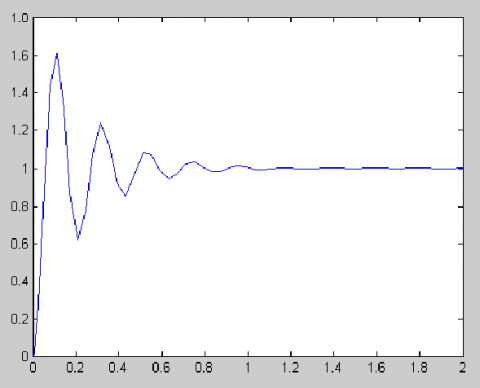Figure 7. The simulation results on the trigonometric function control

The results show that a large position overshoot occurred during the positioning, only relying on the trigonometric function control. The simulation results on feedforward control are shown in Figure 8.

## 8.png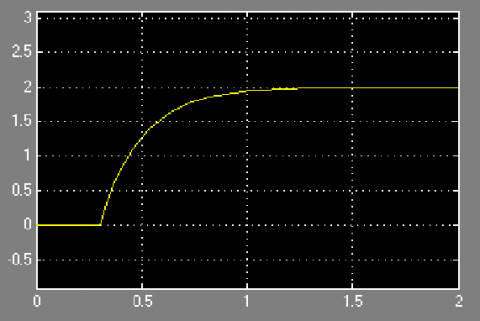Figure 8. The simulation results on feedforward control

As shown in Figure 8, it is obvious that the feedforward control alone only eliminated the position overshoot, but failing to speed up the dynamic responses.

The simulation results on feedforward control combined with trigonometric function control is displayed in Figure 9.

## 9.png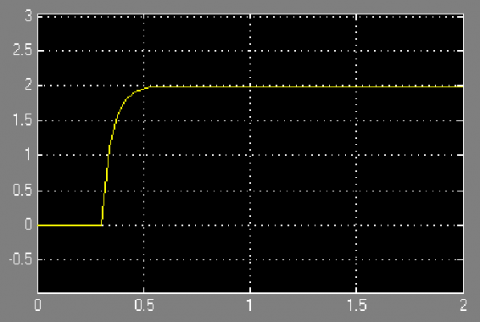Figure 9. The simulation results on feedforward control combined with trigonometric function control

Figure 9 shows that the combined algorithm realized fast positioning without any position overshoot.

4. Conclusions

The semi-automatic production line of cracked connecting rods is a complex and high-precision manufacturing system. This paper attempts to achieve the automatic control of the production line. Firstly, the relevant control theories and techniques were reviewed in details. Next, a precise position control algorithm was designed for the walking beam manipulator under the electric drive mode. The simulation experiments show that the proposed algorithm can achieve precise position control of the manipulator and different workstations, during the transmission of the connecting rods.

References

 Wang, H.Y., Pan, C.H. (2013). On the application and the development trend of mechatronics technology. Advanced Materials Research, 717: 895-898. https://doi.org/10.4028/www.scientific.net/AMR.717.895

 Wu, F. (2014). Cement raw mill based on PCS7 and PROFIBUS production line automation control system. Applied Mechanics and Materials, 644-650: 722-725. https://doi.org/10.4028/www.scientific.net/AMM.644-650.722

 Zhang, Z.S., Wang, Y., Wang, C.S., Cui, J., Liu, Z.Q. (2014). The control of high voltage electrostatic precipitator based on EDA. Advanced Materials Research, 910: 336-339. https://doi.org/10.4028/www.scientific.net/AMR.910.336

 Hara, S., Yamamoto, Y., Omata, T., Nakano, M. (2002). Repetitive control system: a new type servo system for periodic exogenous signals. IEEE Transactions on Automatic Control, 33(7): 659-668. https://doi.org/10.1109/9.1274

 Wang, A., Lin, S. (2011). A variable structure control for electrohydraulic position servo systems with a nonlinear plant model. Journal of the Chinese Institute of Engineers, 14(2): 215-222. https://doi.org/10.1080/02533839.1991.9677327

 Chen, H.M., Renn, J.C., Su, J.P. (2005). Sliding mode control with varying boundary layers for an electro-hydraulic position servo system. International Journal of Advanced Manufacturing Technology, 26(1-2): 117-123. https://doi.org/10.1007/s00170-004-2145-0

 Ba, D.B., Uchiyama, N., Simba, K.R. (2016). Contouring control for three-axis machine tools based on nonlinear friction compensation for lead screws. International Journal of Machine Tools & Manufacture, 108: 95-105. https://doi.org/10.1016/j.ijmachtools.2016.06.001

 Zhang, L., Wang, X., Cao J. (2014). Measurement and compensation of pitch error based on GMA with elimination of its hysteresis. Journal of Mechanical Science and Technology, 28(5): 1855-1866. https://doi.org/10.1007/s12206-014-0333-x

 Zhou, L., Zhao, G. (2008). Application of fuzzy-PID control algorithm in uniform velocity temperature control system of resistance furnace. Chinese Journal of Scientific Instrument, 29(2): 405-409. https://doi.org/10.1080/00207540801918588

 Ge, L.I., Jia, Y., Zhang, H., Zhao, Y. (2008). Application of integral-separation PID control algorithm in PLC-based tension control system. Journal of Textile Research, 29(8): 109-112. https://doi.org/10.3321/j.issn:0253-9721.2008.08.029

 Godbolt, B., Vitzilaios, N.I., Lynch, A.F. (2013). Experimental validation of a helicopter autopilot design using model-based PID control. Journal of Intelligent & Robotic Systems, 70(1-4): 385-399. https://doi.org/10.1007/s10846-012-9720-7

 Liacu, B., Koru, A.T., Ozbay, H., (2012). Low-order controller design for haptic systems under delayed feedback. Ifac Proceedings Volumes, 45(14): 43-48. https://doi.org/10.3182/20120622-3-us-4021.00049

 Wang, Q.G., Zhang, Z., Astrom, K.J., (2009). Guaranteed dominant pole placement with PID controllers. Journal of Process Control, 19(2): 349-352. https://doi.org/10.1016/j.jprocont.2008.04.012

 Shu, H., Shu, H.L. (2006). Development and simulation of PID neural networks controller based on LabView. Journal of System Simulation, 18(10): 2918-2920. https://doi.org/10.3969/j.issn.1004-731X.2006.10.056

 Yang, C.G., Yang, Z.J., Chen, F., (2013). The optimization of the PID controller using the least squares method and the improved Lbest PSO algorithm. Applied Mechanics & Materials, 397-400: 1296-1303. https://doi.org/10.4028/www.scientific.net/amm.397-400.1296

 Li, D., Li, Z., Gao, Z., (2014). Active disturbance rejection-based high-precision temperature control of a semibatch emulsion polymerization reactor. Industrial & Engineering Chemistry Research, 53(8): 3210-3221. https://doi.org/10.1021/ie402544n

 Yu, H., Cheng, S., Zhou, D. (2013). The design and simulation of networked control systems with online extreme learning machine PID. International Journal of Modelling Identification & Control, 20(4): 337-343. https://doi.org/10.1504/ijmic.2013.057567